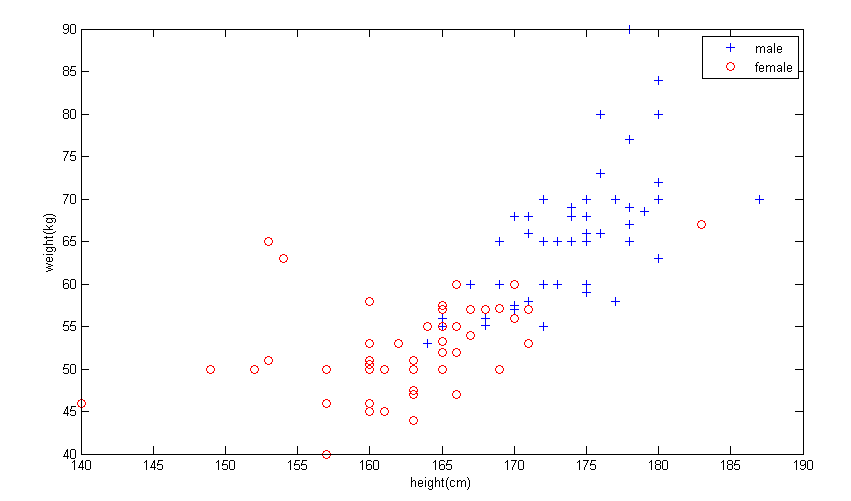# 朴素贝叶斯法

## 条件独立性假设

$P(X=x|Y=c_k)=P(X^{(1)}=x^{(1)},\ldots,X^{(n)}=x^{(n)}|Y=c_k), k=1,2,\ldots,K$

$P(X=x|Y=c_k)$

$=P(X^{(1)}=x^{(1)},\ldots,X^{(n)}=x^{(n)}|Y=c_k)$

$=\prod^n_{j=1} P(X^{(j)}|Y=c_k)$

## 贝叶斯定理

$P(Y|X) = \frac{P(X,Y)}{P(X)}=\frac{P(Y)P(X|Y)}{\sum_YP(P(Y)P(X|Y))}$

$y=\arg \max_{c_k}P(Y=c_k)P(X_j=x^{(j)}|Y=c_k)$

$y=\arg \max_{c_k}P(Y=c_k) \prod_j P(X_j=x^{(j)}|Y=c_k)$

# 朴素贝叶斯法的参数估计

## 极大似然估计

$P(Y=c_k)=\frac{\sum^N_i I(y_i=c_i)}{N},k=1,2,\ldots,K$

# 应用实例

## 数据# 参考文献

1. 李航. 统计学习方法.清华大学出版社.2012